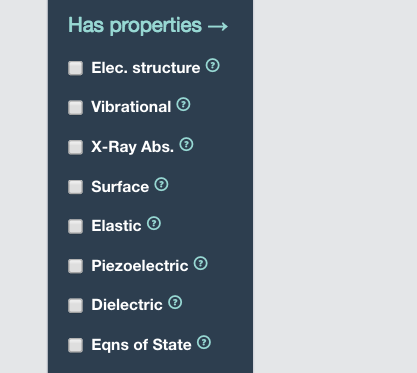# Mp-571183 TmFe2 DOS & Band Strucutre

Dear Experts

I would like to ask a very simple questions regarding mp-571183 TmFe2

My questions are 1- The Final Magnetic Moment 6.244 μB is this result per formula unit or not

2- I would like to ask if there are available results for DOS & Band structure for this computed TmFe2

Thank You

To answer your first question, the total magnetisation is per unit cell, so it is not necessarily normalized per formula unit at present. We may change this to be per formula unit in future, but will communicate this change clearly if so.

1 Like

Hello mkhorton

Thank you v. much for your quick answer for my first question (Means I have to divide by 2 to be per formula unit)